## Mandelbrot 2019 Calendar images

Mandelbrot Explorer program
that I am using for a 2019 calendar.
(A copy of the calendar can be purchased from Lulu.com.)

The table below shows small versions of the images for
January through December. The second column contains the
x and y limits on the region of the plane that is shown
in the image, and the number of iterations that were used.
The third column contains three links:
a link to the full-size, 300 dot per inch image which is
used for the calendar; a link to a 1/3 size image that will be
11-by-8.5 inches at 100 dots per inch; and a link to the
Mandelbrot Explorer app that will open the example in that
app, so that you can zoom in and out, change the colors,
and play with the image in other ways.

Also available:  2017 calendar images  and  2018 calendar images.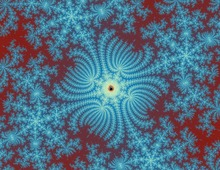xmin = -1.244130845439453111212
xmax = -1.244130819033203111212
ymin = 0.072542285392246685544
ymax = 0.072542305797076230998
max iterations = 20000
Large Image (3300-by-2550)

Small Image (1100-by-850)

Open in Mandelbrot Explorer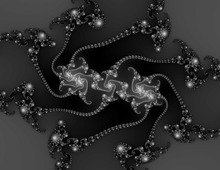xmin = 0.300792861590402533020
xmax = 0.300792861728340278228
ymin = 0.020152732375044561157
ymax = 0.020152732481632818817
max iterations = 2500
Large Image (3300-by-2550)

Small Image (1100-by-850)

Open in Mandelbrot Explorer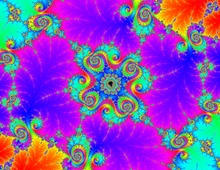xmin = -0.109329365789381977958
xmax = -0.109329365635221013840
ymin = 0.894818654783724217041
ymax = 0.894818654902848598405
max iterations = 150000
Large Image (3300-by-2550)

Small Image (1100-by-850)

Open in Mandelbrot Explorer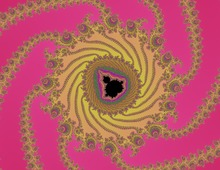xmin = -0.7243497379314300930650
xmax = -0.7243497379308410190090
ymin = 0.1847237708752381324566
ymax = 0.1847237708756933260454
max iterations = 10000
Large Image (3300-by-2550)

Small Image (1100-by-850)

Open in Mandelbrot Explorer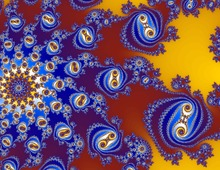xmin = -0.169935537190244337077
xmax = -0.169934494864621883577
ymin = 0.657928447556851934600
ymax = 0.657929252990287466850
max iterations = 5000
Large Image (3300-by-2550)

Small Image (1100-by-850)

Open in Mandelbrot Explorer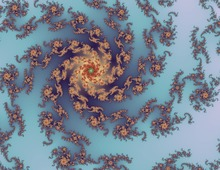xmin = -0.883802883773486103663
xmax = -0.883802879856255172431
ymin = 0.235318188960411083907
ymax = 0.235318191987362258041
max iterations = 2500
Large Image (3300-by-2550)

Small Image (1100-by-850)

Open in Mandelbrot Explorerxmin = -1.624431520283920000320
xmax = -1.624431520107744368320
ymin = 0.002531244656254482832
ymax = 0.002531244792390198468
max iterations = 5000
Large Image (3300-by-2550)

Small Image (1100-by-850)

Open in Mandelbrot Explorer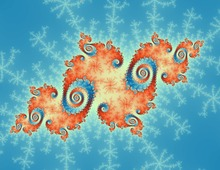xmin = -0.691472729826910634752
xmax = -0.691472729809904908352
ymin = 0.465703143529837884183
ymax = 0.465703143542978672765
max iterations = 5000
Large Image (3300-by-2550)

Small Image (1100-by-850)

Open in Mandelbrot Explorer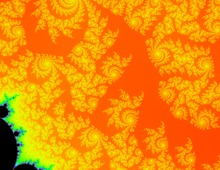xmin = 0.354719310971257600000
xmax = 0.354740992684220550000
ymin = 0.095401756110146627046
ymax = 0.095418510161072542954
max iterations = 75000
Large Image (3300-by-2550)

Small Image (1100-by-850)

Open in Mandelbrot Explorerxmin = -1.674409673704940858567
xmax = -1.674409673685731820699
ymin = 0.000047165618655634221
ymax = 0.000047165633498981665
max iterations = 25000
Large Image (3300-by-2550)

Small Image (1100-by-850)

Open in Mandelbrot Explorer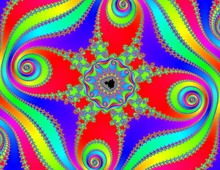xmin = -0.753231765876289000000
xmax = -0.753231756503881100000
ymin = 0.046335503033396827273
ymax = 0.046335510275712022727
max iterations = 15000
Large Image (3300-by-2550)

Small Image (1100-by-850)

Open in Mandelbrot Explorerxmin = 0.347610822323866892630
xmax = 0.347610822324533812266
ymin = 0.084679408736920746723
ymax = 0.084679408737436093715
max iterations = 10000
Large Image (3300-by-2550)

Small Image (1100-by-850)

Open in Mandelbrot Explorer(Cover) Large Image (3300-by-2550)

Small Image (1100x850)